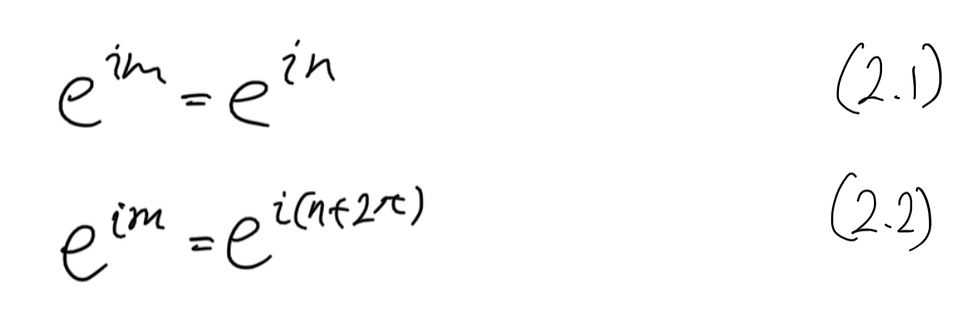top of page
All Posts: Blog2

# [Math Evenings] Banach-Tarski Revisited 2: 2D Paradox

As a warm-up before the Banach-Tarski Paradox, we will prove a theorem that is the 2D version of the Banach-Tarski Paradox.

As I mentioned before, the greatness of the Banach Tarski lies in not only its elegance, but also the fact that we can produce two spheres from one by dividing the original sphere up into a finite number of pieces and a finite number of rigid motions. If we were able to split the sphere up into infinitely many pieces, there is no problem in grinding the original sphere into basically a powder of each particle having an infinitesimal radius, and restructuring it to form two spheres. But the very fact that we use a finite number of pieces and a finite number of rigid motions is what makes the theorem so magnificently beautiful.

The theorem below is hence a crude and more elementary of the Banach-Tarski Paradox.

Theorem 1.

For a unit circle C on the imaginary plane, consider C-{1} by excluding a point z=1 from C. Then, we can partition the unit circle into two areas A, B, and rotate B to obtain B' such that the union of A and B' is C.

Pf.

First, let us define the area B as eq 1.Then, for m and n defined as eq 2.1, eq 2.2 must also hold true. However, note that the power of the left hand side in eq 2.2 is not a natural number.Hence, all elements in B are distinct elements with one another, and are elements of the unit circle C. Then, A can be defined as eq 3.1. Note here that the set of natural numbers is a countably infinite set, and hence B is also a countably infinite set. This would mean that the union of A and B would be C-{1} as in eq 3.2.Now we will apply rigid motion as in eq 4 to the set B.This in turn gives us eq 5. But note that when n=1, 1 becomes an element of B' as eq 6 tells us.Hence, from the definition of set A, the union of A and B' is C.Q.E.D.

I think that this proof is one of the most straightforward and at the same time, not straightforward proofs. There is no complicated mathematics involved, but it gives us a surprising result that infinity plus one equals infinity. I personally believe this theorem to be a geometric representation of Hilbert's hotel paradox.

25 views

See All
bottom of page